# Canon Science Lab

## Light is It a Wave or a Particle?

Just what is the true nature of light? Is it a wave or perhaps a flow of extremely small particles? These questions have long puzzled scientists. Let's travel through history as we study the matter.

Around 1700, Newton concluded that light was a group of particles (corpuscular theory). Around the same time, there were other scholars who thought that light might instead be a wave (wave theory). Light travels in a straight line, and therefore it was only natural for Newton to think of it as extremely small particles that are emitted by a light source and reflected by objects. The corpuscular theory, however, cannot explain wave-like light phenomena such as diffraction and interference. On the other hand, the wave theory cannot clarify why photons fly out of metal that is exposed to light (the phenomenon is called the photoelectric effect, which was discovered at the end of the 19th century). In this manner, the great physicists have continued to debate and demonstrate the true nature of light over the centuries.

### Light Is a Particle! (Sir Isaac Newton)

Known for his Law of Universal Gravitation, English physicist Sir Isaac Newton (1643 to 1727) realized that light had frequency-like properties when he used a prism to split sunlight into its component colors. Nevertheless, he thought that light was a particle because the periphery of the shadows it created was extremely sharp and clear.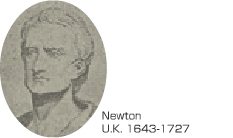### Light Is a Wave! (Grimaldi and Huygens)

The wave theory, which maintains that light is a wave, was proposed around the same time as Newton's theory. In 1665, Italian physicist Francesco Maria Grimaldi (1618 to 1663) discovered the phenomenon of light diffraction and pointed out that it resembles the behavior of waves. Then, in 1678, Dutch physicist Christian Huygens (1629 to 1695) established the wave theory of light and announced the Huygens' principle.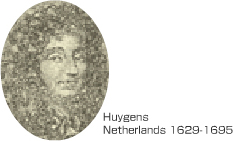### Light Is Unequivocally a Wave! (Fresnel and Young)

Some 100 years after the time of Newton, French physicist Augustin-Jean Fresnel (1788 to 1827) asserted that light waves have an extremely short wavelength and mathematically proved light interference. In 1815, he devised physical laws for light reflection and refraction, as well. He also hypothesized that space is filled with a medium known as ether because waves need something that can transmit them. In 1817, English physicist Thomas Young (1773 to 1829) calculated light's wavelength from an interference pattern, thereby not only figuring out that the wavelength is 1 μm ( 1 μm = one millionth of a meter ) or less, but also having a handle on the truth that light is a transverse wave. At that point, the particle theory of light fell out of favor and was replaced by the wave theory.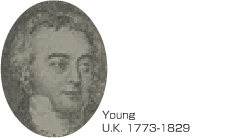### Light Is a Wave - an Electromagnetic Wave! (Maxwell)

The next theory was provided by the brilliant Scottish physicist James Clerk Maxwell (1831 to 1879). In 1864, he predicted the existence of electromagnetic waves, the existence of which had not been confirmed before that time, and out of his prediction came the concept of light being a wave, or more specifically, a type of electromagnetic wave. Until that time, the magnetic field produced by magnets and electric currents and the electric field generated between two parallel metal plates connected to a charged capacitor were considered to be unrelated to one another. Maxwell changed this thinking when, in 1861, he presented Maxwell's equations: four equations for electromagnetic theory that shows magnetic fields and electric fields are inextricably linked. This led to the introduction of the concept of electromagnetic waves other than visible light into light research, which had previously focused only on visible light.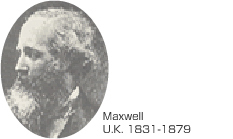The term electromagnetic wave tends to bring to mind the waves emitted from cellular telephones, but electromagnetic waves are actually waves produced by electricity and magnetism. Electromagnetic waves always occur wherever electricity is flowing or radio waves are flying about. Maxwell's equations, which clearly revealed the existence of such electromagnetic waves, were announced in 1861, becoming the most fundamental law of electromagnetics. These equations are not easy to understand, but let's take an in-depth look because they concern the true nature of light.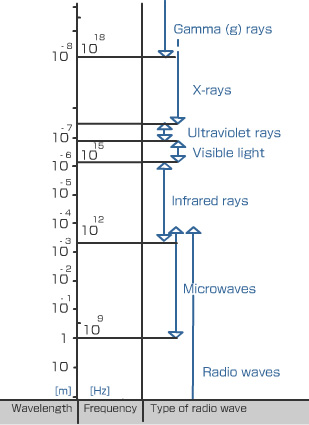### What Are Maxwell's Equations?

Maxwell's four equations have become the most fundamental law in electromagnetics. The first equation formulates Faraday's Law of Electromagnetic Induction, which states that changing magnetic fields generate electrical fields, producing electrical current.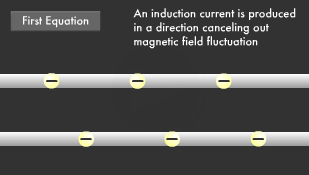The second equation is called the Ampere-Maxwell Law. It adds to Ampere's Law, which states an electric current flowing over a wire produces a magnetic field around itself, and another law that says a changing magnetic field also gives rise to a property similar to an electric current (a displacement current), and this too creates a magnetic field around itself. The term displacement current actually is a crucial point.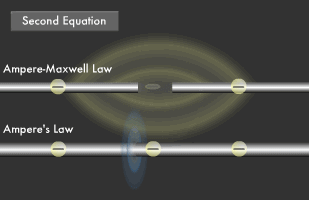The third equation is the law stating there is an electric charge at the source of an electric field.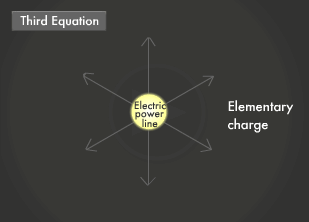The fourth equation is Gauss's Law of magnetic field, stating a magnetic field has no source (magnetic monopole) equivalent to that of an electric charge.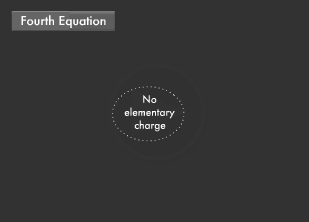### What is Displacement Current?

If you take two parallel metal plates (electrodes) and connect one to the positive pole and the other to the negative pole of a battery, you will create a capacitor. Direct-current (DC) electricity will simply collect between the two metal plates, and no current will flow between them. However, if you connect alternating current (AC) that changes drastically, electric current will start to flow along the two electrodes. Electric current is a flow of electrons, but between these two electrodes there is nothing but space, and thus electrons do not flow.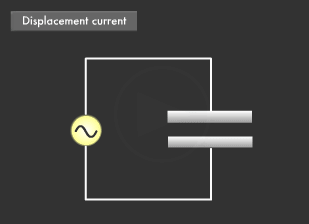Maxell wondered what this could mean. Then it came to him that applying an AC voltage to the electrodes generates a changing electric field in the space between them, and this changing electric field acts as a changing electric current. This electric current is what we mean when we use the term displacement current.

### What Are Electromagnetic Waves and Electromagnetic Fields?

A most unexpected conclusion can be drawn from the idea of a displacement current. In short, electromagnetic waves can exist. This also led to the discovery that in space there are not only objects that we can see with our eyes, but also intangible fields that we cannot see. The existence of fields was revealed for the first time. Solving Maxwell's equations reveals the wave equation, and the solution for that equation results in a wave system in which electric fields and magnetic fields give rise to each other while traveling through space.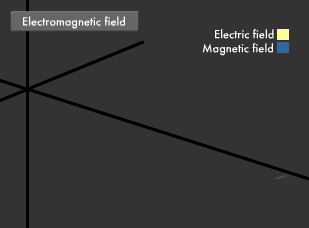The form of electromagnetic waves was expressed in a mathematical formula. Magnetic fields and electric fields are inextricably linked, and there is also an entity called an electromagnetic field that is solely responsible for bringing them into existence.

### What Is the Principle Behind Electromagnetic Wave Generation?

Now let's take a look at a capacitor. Applying AC voltage between two metal electrodes produces a changing electric field in space, and this electric field in turn creates a displacement current, causing an electric current to flow between the electrodes. At the same time, the displacement current produces a changing magnetic field around itself according to the second of Maxwell's equations (Ampere-Maxwell Law).The resulting magnetic field creates an electric field around itself according to the first of Maxwell's equations (Faraday's Law of Electromagnetic Induction). Based on the fact that a changing electric field creates a magnetic field in this manner, electromagnetic waves-in which an electric field and magnetic field alternately appear-are created in the space between the two electrodes and travel into their surroundings. Antennas that emit electromagnetic waves are created by harnessing this principle.

### How Fast Are Electromagnetic Waves?

Maxwell calculated the speed of travel for the waves, i.e. electromagnetic waves, revealed by his mathematical formulas. He said speed was simply one over the square root of the electric permittivity in vacuum times the magnetic permeability in vacuum. When he assigned "9 x 109/4π for the electric permittivity in vacuum" and "4π x 10-7 for the magnetic permeability in vacuum," both of which were known values at the time, his calculation yielded 2.998 x 108 m/sec. This exactly matched the previously discovered speed of light. This led Maxwell to confidently state that light is a type of electromagnetic wave.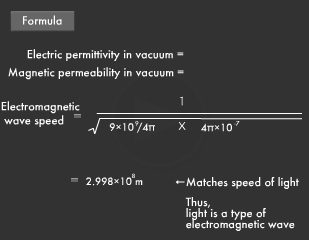### Light Is Also a Particle! (Einstein)

The theory of light being a particle completely vanished until the end of the 19th century when Albert Einstein revived it. Now that the dual nature of light as "both a particle and a wave" has been proved, its essential theory was further evolved from electromagnetics into quantum mechanics. Einstein believed light is a particle (photon) and the flow of photons is a wave. The main point of Einstein's light quantum theory is that light's energy is related to its oscillation frequency. He maintained that photons have energy equal to "Planck's constant times oscillation frequency," and this photon energy is the height of the oscillation frequency while the intensity of light is the quantity of photons. The various properties of light, which is a type of electromagnetic wave, are due to the behavior of extremely small particles called photons that are invisible to the naked eye.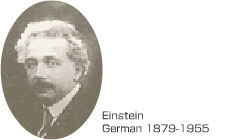### What Is the Photoelectric Effect?

The German physicist Albert Einstein (1879 to 1955), famous for his theories of relativity, conducted research on the photoelectric effect, in which electrons fly out of a metal surface exposed to light. The strange thing about the photoelectric effect is the energy of the electrons (photoelectrons) that fly out of the metal does not change whether the light is weak or strong. (If light were a wave, strong light should cause photoelectrons to fly out with great power.) Another puzzling matter is how photoelectrons multiply when strong light is applied. Einstein explained the photoelectric effect by saying that "light itself is a particle," and for this he received the Nobel Prize in Physics.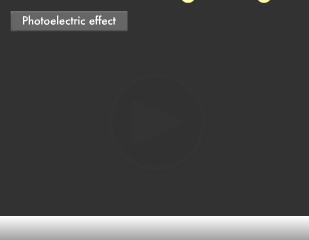### What Is a Photon?

The light particle conceived by Einstein is called a photon. The main point of his light quantum theory is the idea that light's energy is related to its oscillation frequency (known as frequency in the case of radio waves). Oscillation frequency is equal to the speed of light divided by its wavelength. Photons have energy equal to their oscillation frequency times Planck's constant. Einstein speculated that when electrons within matter collide with photons, the former takes the latter's energy and flies out, and that the higher the oscillation frequency of the photons that strike, the greater the electron energy that will come flying out.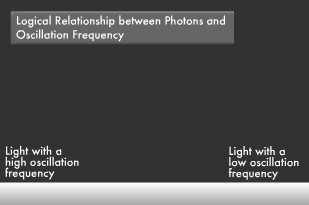In short, he was saying that light is a flow of photons, the energy of these photons is the height of their oscillation frequency, and the intensity of the light is the quantity of its photons.

Einstein proved his theory by proving that the Planck's constant he derived based on his experiments on the photoelectric effect exactly matched the constant 6.6260755 x 10-34 (Planck's constant) that German physicist Max Planck (1858 to 1947) obtained in 1900 through his research on electromagnetic waves. This too pointed to an intimate relationship between the properties and oscillation frequency of light as a wave and the properties and momentum (energy) of light as a particle, or in other words, the dual nature of light as both a particle and a wave.### Do Other Particles Besides Photons Become Waves?

French theoretical physicist Louis de Broglie (1892 to 1987) furthered such research on the wave nature of particles by proving that there are particles (electrons, protons and neutrons) besides photons that have the properties of a wave. According to de Broglie, all particles traveling at speeds near that of light adopt the properties and wavelength of a wave in addition to the properties and momentum of a particle. He also derived the relationship "wavelength x momentum = Planck's constant."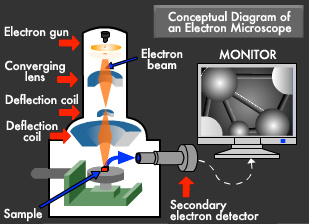From another perspective, one could say that the essence of the dual nature of light as both a particle and a wave could already be found in Planck's constant. The evolution of this idea is contributing to diverse scientific and technological advances, including the development of electron microscopes.